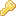Log in

Patch fürs VotePlugin

08 Feb 2013 - 13:58 | Version 1 |

Quelle: http://twiki.org/cgi-bin/view/Plugins/VotePluginDev

Grund: Damit er die einzelnen Umfragen einzeln wertet, auch wenn alle mit `saveto` ans gleiche ort gespeichert werden.
```--- Core.pm.orig        2008-03-04 08:41:23.000000000 +0000
+++ Core.pm     2008-03-04 08:44:36.000000000 +0000
@@ -167,22 +167,22 @@
my %totalVoters;  # how many different people voted for each key
my %totalRate;    # Total of all ratings for each key
my %items;        # Hash of id's that have the same key
-    my \$voteSum = 0;  # Sum of the number of votes on all rated items
-    my \$rateSum = 0;  # Sum of all ratings of rated items
+    my %voteSum;      # Sum of the number of votes on all rated items
+    my %rateSum;      # Sum of all ratings of rated items
foreach my \$voter (keys %votes) {
foreach my \$vid (keys %{\$votes{\$voter}}) {
foreach my \$key (keys %{\$votes{\$voter}{\$vid}}) {
\$keyValueFreq{\$vid}{\$key}{\$choice} += \$weight;
\$items{\$key}{\$vid} = 1;
if (\$choice =~ /^[\d.]+\$/) {
-                    \$totalRate{\$key} += \$choice * \$weight;
-                    \$rateSum += \$choice * \$weight;
+                    \$totalRate{\$key}{\$vid} += \$choice * \$weight;
+                    \$rateSum{\$key} += \$choice * \$weight;
}
-                \$totalVoters{\$key}++;
+                \$totalVoters{\$key}{\$vid}++;
}
}
}
@@ -218,22 +218,22 @@
# avg_rating: The average rating of each item (again, of those that
my \$myLastVote =
my \$mean = 0;
-                \$mean = \$totalRate{\$key} / \$totalVotes{\$key};
+                \$mean = \$totalRate{\$key}{\$id} / \$totalVotes{\$key}{\$id};
if (\$bayesian) {
\$mean = (\$avg_num_votes * \$avg_rating +